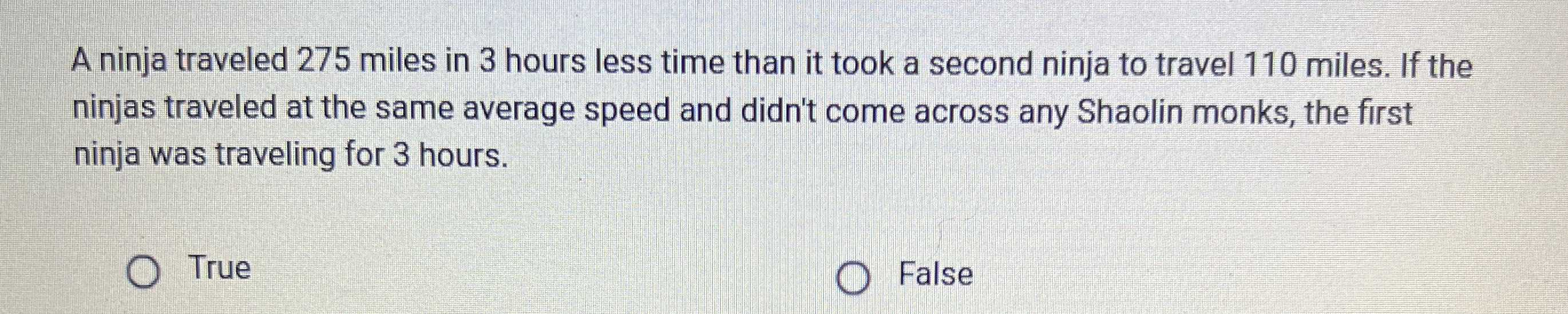### ¿Todavía tienes preguntas de matemáticas?

Pregunte a nuestros tutores expertos
Algebra
PreguntaA ninja traveled $$275$$ miles in $$3$$ hours less time than it took a second ninja to travel $$110$$ miles. If the ninjas traveled at the same average speed and didn't come across any Shaolin monks, the first ninja was traveling for $$3$$ hours.

$$\ false$$